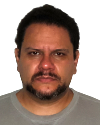#Wolfram Summer School

# Alumni# Jorge Villalobos

Educational Innovation

Class of 2016

## Bio

I was born in 1976, and I’m a physicist with an MsC in physics and a doctorate in engineering (both from Universidad de los Andes in Colombia). I’m currently the dean of the Faculty of Natural Sciences and Mathematics at Universidad de Ibagué (Colombia). My research interests are in nonlinear systems. I like horseback riding, rugby, pets and reading.

## Project: Explorations for Basic Skills

Is there a heuristic to build or identify challenging problems for university-level, mathematics-related courses that involve the student in doing computation or simulation? The pedagogical model that begs for this question to be answered is one where classical, chalkboard-based teaching is taken out of the classroom. In this model, the student becomes responsible for acquiring the textbook skills, and the classroom is turned into a mathematics laboratory. In this laboratory, students are posed challenging problems that force them to use mathematics, computer simulation, critical thinking and other general competencies like teamwork.

A challenging problem should have incomplete (or an excess of) information, may possibly require the gathering of data to work it out and should not have a unique answer. Ideally, all known solutions to the problem are somehow flawed. I am thinking of complex problems, understood as problems where we know that the answer exists, but coming up with it is not trivial.

In a precalculus course at Universidad de Ibagué (4 hours a week for 14 weeks—a workload of 168 hours for the student), the topics are organized as follows:

• Set theory (1 week)
• Real numbers (sets, properties, addition, …, powers, roots, log) (2 weeks)
• Basic algebra (linear systems, inequalities) (3 weeks)
• Functions (3 weeks)
• Basic geometry (3 weeks)
• Trigonometry (1 week)
• Exams (1 week)

I propose focusing on set theory and real numbers. The main objective would be to make the course computer based. This is to develop a set of explorations that lead to challenging problems that involve the use of computational thinking, basic programming and concepts of set theory.

In order to make the computer-based approach, a sufficient introduction to the Wolfram Language vocabulary and syntax is mandatory; therefore, the explorations should introduce both mathematical and Wolfram Language concepts.

• 21st Annual Wolfram Summer School
• Bentley University, Waltham, MA, USA
• June 25–July 15, 2023